# Issues Related to Pile Tile Grillage Calculation

Temuri Kikava*

Batumi Shota Rustaveli State University, Georgia

Received Date: July 25, 2019;  Published Date: July 31, 2019

#### Abstract

Taking the general provisions of the theory of prof. I Simvulidi as a basis and applying a model of a linearly deformable half-space to the ground, the tile grillage is mentally imagined as a system of mutually intersecting bands relying on stanchions and on the ground. The formulas for calculation are given below. A specific engineering problem is considered.

Keywords: Flexibility rate; Tile grillage; Linear stiffness; Pile-racks; System of linear equations; Soil reaction

#### Introduction

The low pile grillages in the form of slabs are widely used in construction practice. Such plates rest partly on the heads of the piles, partly on the soil surrounding the piles, and distribute the total load on the piles and on the foundation. This problem is still insufficiently studied, and it is often considered that the total weight of the structure is transferred to the base by piles, not taking into account the load transfer by the foundation of the grillage or by a solid slab. The purpose of the study was to study the actual work of the tile grillage, considering and calculating it as a system of mutually intersecting bands.

#### The Main Part

Using the deformation compatibility equations at the intersection of the slab strips and the contact condition of the slab and base, we write the equation for the strips intersecting at the nodes 11, 21, ..., m1, 1n, 2n, ..., mn (Figure 1)At the beginning, we compose a system of linear equations for finding the unknown forces Ymn arising at the nodes of the intersection of the lanes in the direction of the axis “0Х”; According to the formula Ymn + Y’mn= Nmn, we find the forces Y’mn acting along the ,,0Z”axis.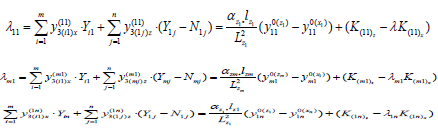Let’s consider as an example a reinforced concrete plate grillage, supported along a contour on a pile-rack. The design diagram of the plate and its geometrical dimensions are given in Fig. 2. Plate thickness h = 20 cm grade of concrete B-20. The modulus of elasticity is E- 20 000 MPa, Poisson’s ratio μ0 = 0.3. Due to the fact that the plate is loaded with symmetrically located loads on it, for its calculation it is enough to consider and calculate its 1/4 part [1,2].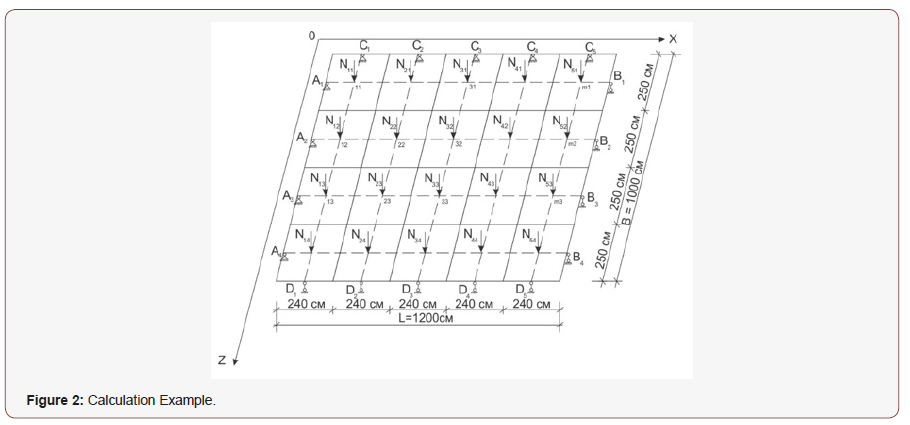The system of linear equations in this case will have the following form: (Figure 2)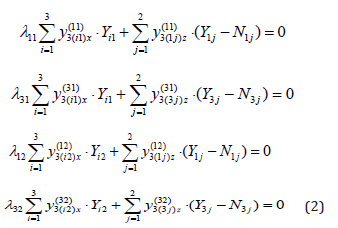The index of flexibility α is determined by the formula: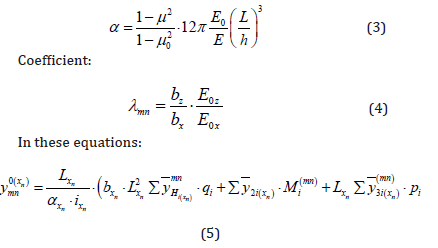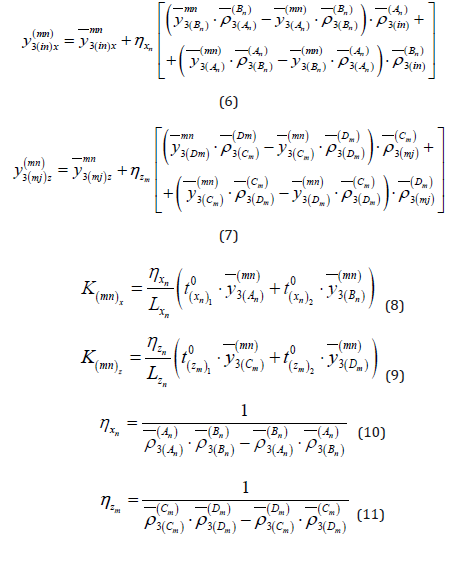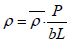- alues of soil reactive pressure;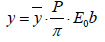- The values of the relative deflections of the beam (lanes);

The valuesandare taken from table  in terms of flexibility α and β.

Reactions from the pile is determined by the formulas: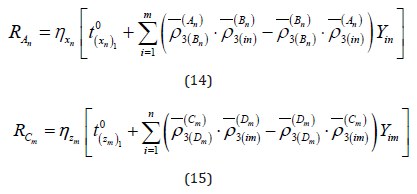wherelinear stiffness of structures.

Let’s determine the β, 𝞴 and α: (β-abstracted abscissa of the place of application of loads)Let’s define ηz1:Let’s define ηx1:Substituting the found numerical values into equations (2) and solving them, we find the unknown forces arising at the intersection points of the lanes in the direction’s “X” and “Z”: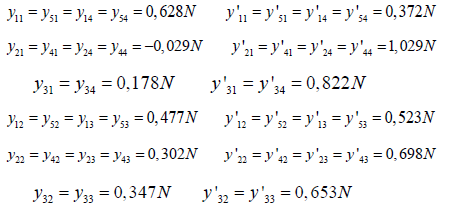Let’s proceed to the definition of reactions in the direction of the axis “X”: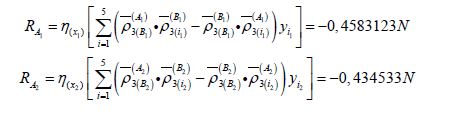In view of the load symmetry: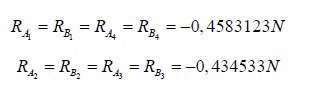Let’s proceed to the definition of reactions in the direction of the axis “Z”: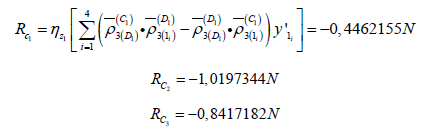In view of the load symmetry:After determining the unknown forces, each band is considered and calculated as a separate one, which lies on a linearly deformable base. In the considered example, 44.4% of the load transferred from the structure, is transferred to the tile grillage, and 55.6% to the piles. Therefore, in such cases, when constructing a tile grillage, we can save materials by reducing the number of piles or their sizes [1-4].

#### Conclusion

According to the proposed method, it is possible to calculate tiled grillages resting on piles and racks, considering them as a system of mutually intersecting bands lying on a linearly deformable base and on piles. Based on the specific engineering problem considered, it can be concluded that in such cases a significant part of the load transferred from the structure assumes a tile grillage, which can lead to significant savings in materials by reducing the number of piles or their sizes.

None.

#### Conflict of Interest

No conflict of interest.

Article Details
Citation
Keywords
Scroll to
Scroll to Top